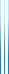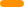# PublicationsPapers

## Bounded indistinguishability for simple sources

Andrej Bogdanov, K. Dinesh, Yuval Filmus, Yuval Ishai, Avi Kaplan, and Akshay Srinivasan
Submitted

Braverman’s celebrated theorem states that $\mathsf{polylog}(n)$-independent sources fool $\mathsf{AC^0}$. In contrast, since the approximate degree of $\mathsf{OR}$ is $\Theta(\sqrt{n})$, we can construct $\Theta(\sqrt{n})$-indistinguishable sources which can be told apart by $\mathsf{OR}$.

In this work, we attempt to bridge this gap by considering what happens when the $k$-indistinguishable sources are simple: have low degree over $\mathbb{F}_2$, or can be sampled in low depth.

Our main positive result constructs $\Theta(\sqrt{n})$-indistinguishable sources distinguishable by $\mathsf{OR}$ which are samplable (a) by polynomial size decision tree (and so by DNFs/CNFs), and (b) by polynomials of degree $O(\log n)$.

In contrast, we show that $O(1)$-indistinguishable sources of constant degree do fool $\mathsf{OR}$, and $O(\log^{10}(n/\epsilon))$-indistinguishable quadratic sources $\epsilon$-fool unambiguous DNFs, even if only one of the two sources is simple. We also show that $O(\log\log(n/\epsilon))$-indistinguishable depth 1 sources are $\epsilon$-close in statistical distance.

## BibTeX

@misc{BDFIKS21,
author = {Andrej Bogdanov and Krishnamoorthy Dinesh and Yuval Filmus and Yuval Ishai and Avi Kaplan and Akshayaram Srinivasan},
title = {Bounded indistinguishability for simple sources},
year = {2021}
}copy to clipboard